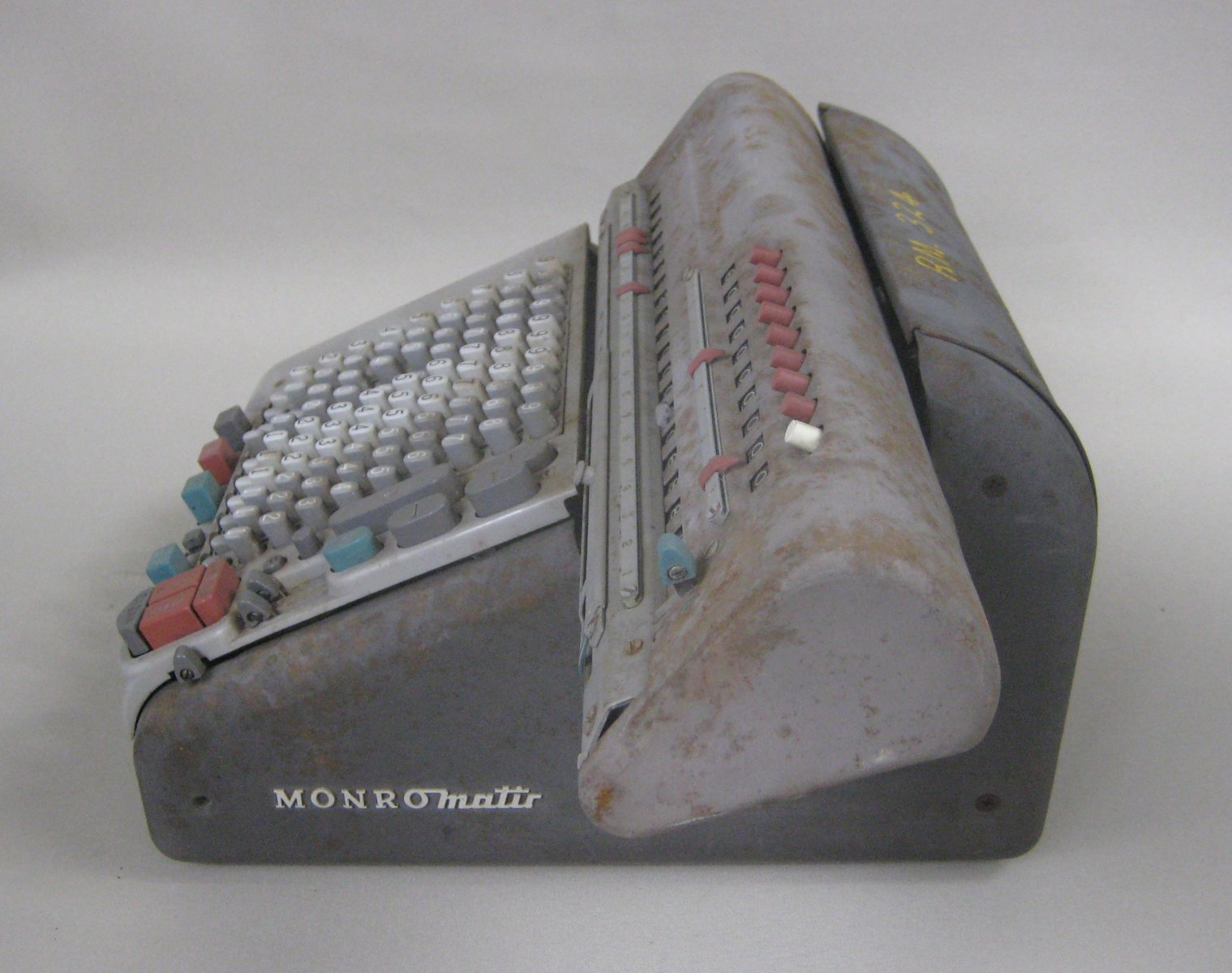ID:
416
Maker's Name:
Monroe
USA
Dimensions:
35 × 29 × 20 cm
Tour Audio:In 1912 founder of Monroe Calculators Jay R. Monroe with calculator designer and partner Frank S. Baldwin introduced the first of many Monroe calculators. These calculators similar to Contex calculators used predominantly decimal scales and complex gearing in order to calculate results.

The user would input on a keyboard the first number to be used in the equation and then the operational handle rotated to add. The second number would be input then and the handle rotated in accordance with addition or subtraction. The operations relied on stepped cylinders and complex stepped gearing to achieve the various operations. Monroe calculators became such a success that progressions such as electric motors, automatic addition, subtraction, multiplication, division and square roots were introduced. The electric motors reduced calculation time and human error significantly. It was not until early 1970’s that the Monroe calculators introduced printer roles and programmability functions to their machines.

As evident with all Monroe’s is their distinct shape due to a sliding upper carriage, similar to a type writer. This carriage allowed the machine, and user, to quickly calculate numbers of higher orders as it gave way to multiplying by different decades. The Monroe calculators soon had fully automated calculators such as the IQ-213 which could compute regular operations as well as square roots and cubes. By the late 1970’s Monroe calculators were becoming so developed that they began introducing ‘pocket’ calculators.

This electrically powered model was used in the UQ Chemistry Department.

This item is part of the UQ Physics Museum Good Old days of Calculation Tour
•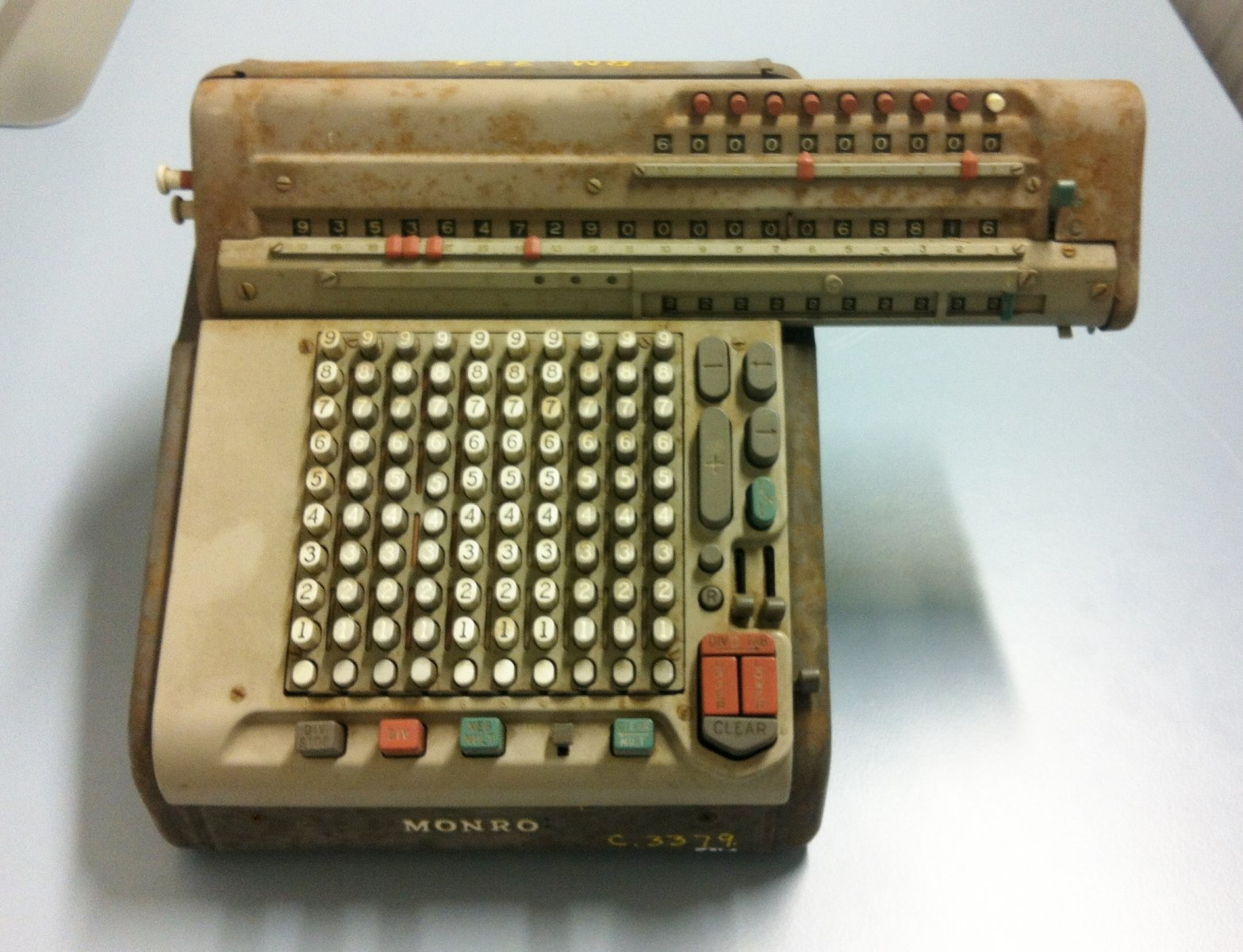•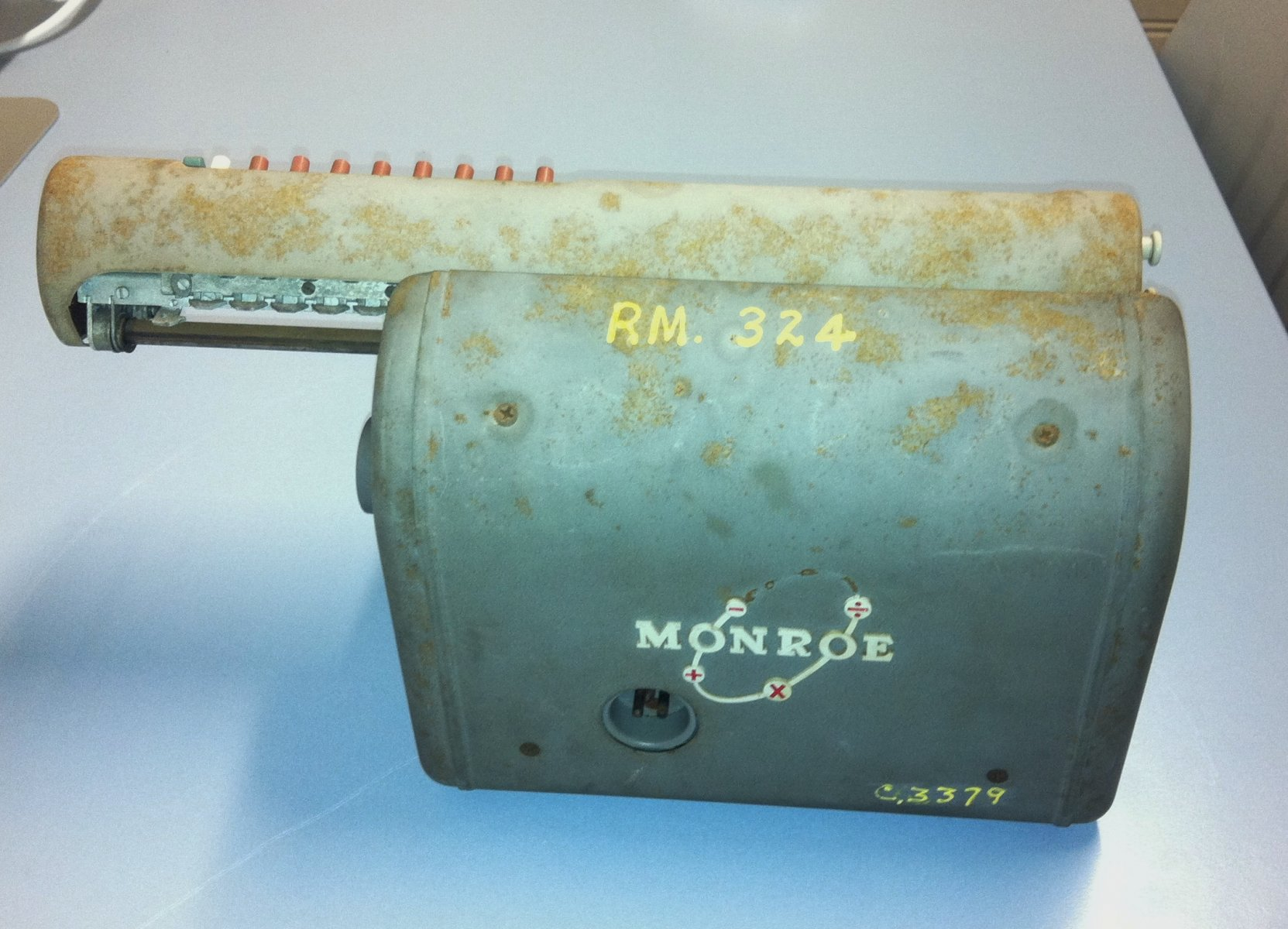••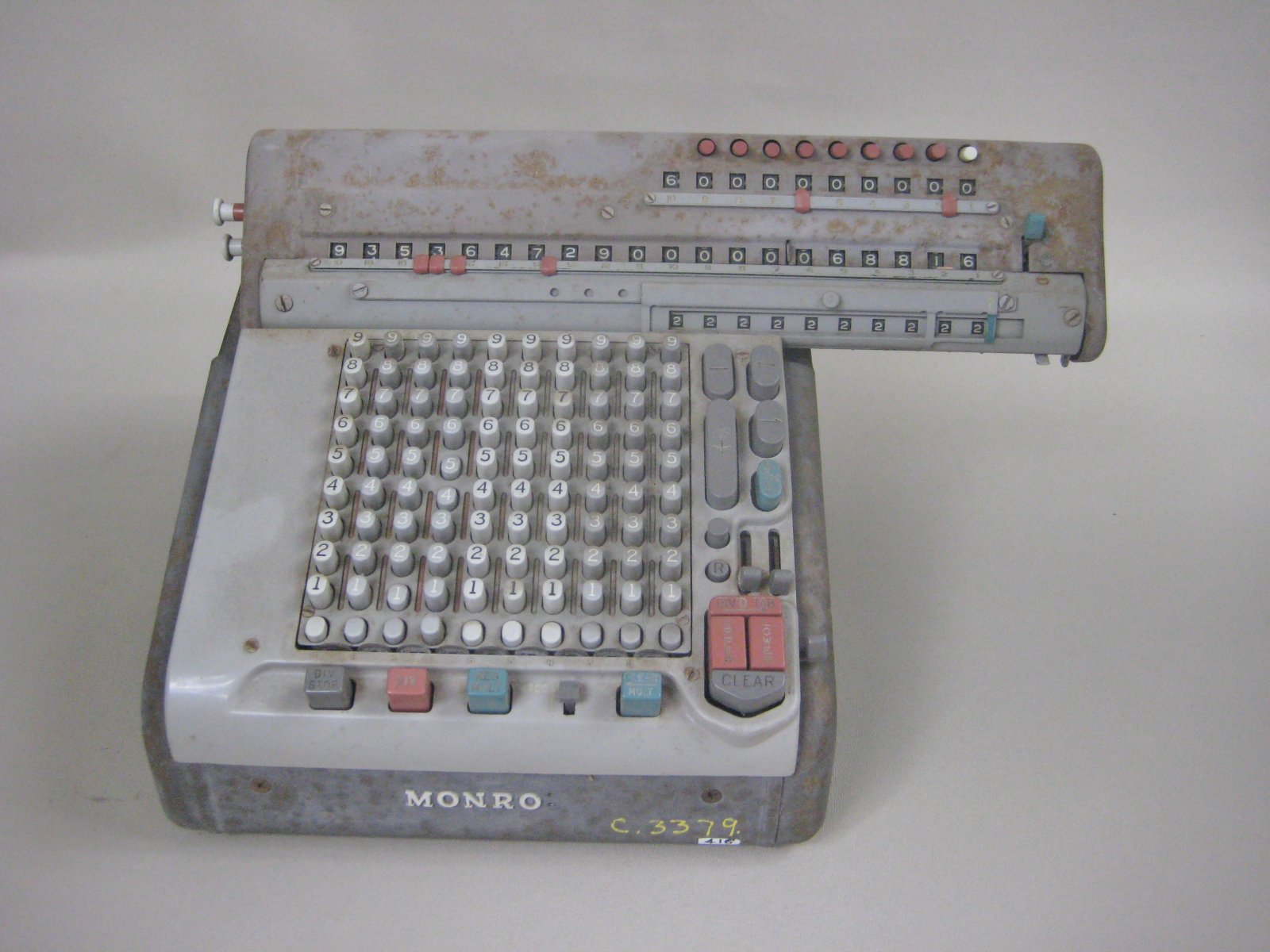•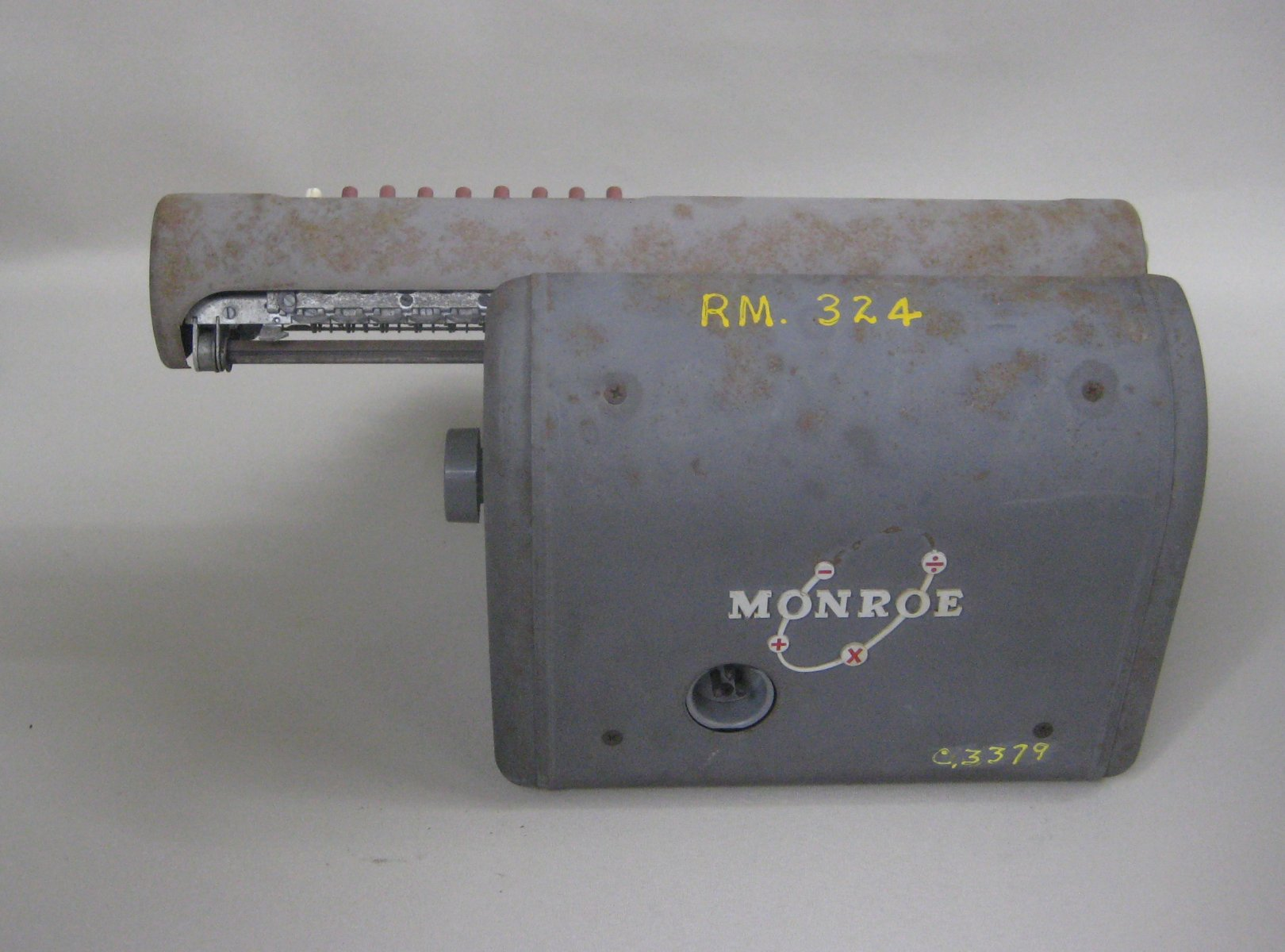•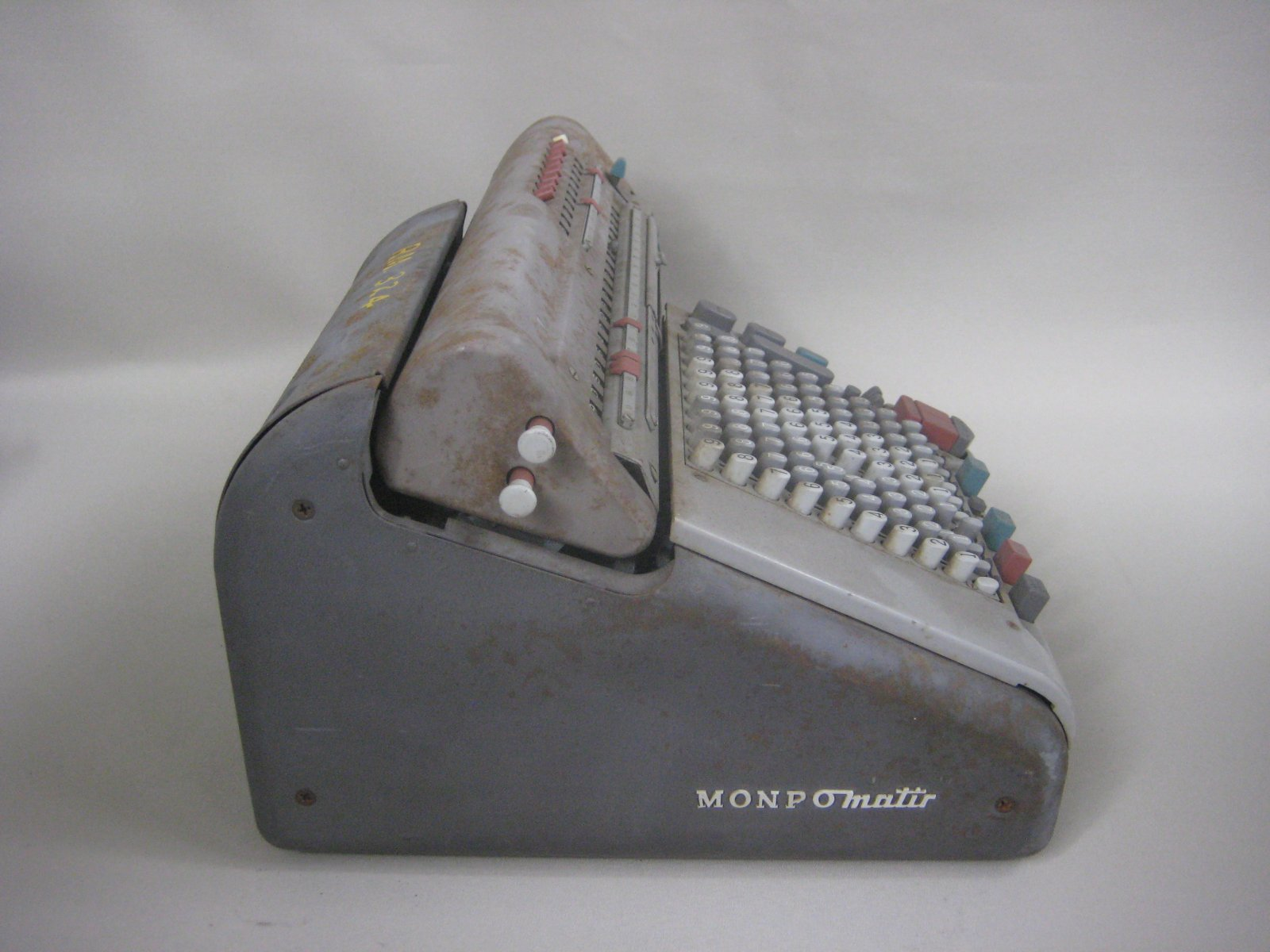•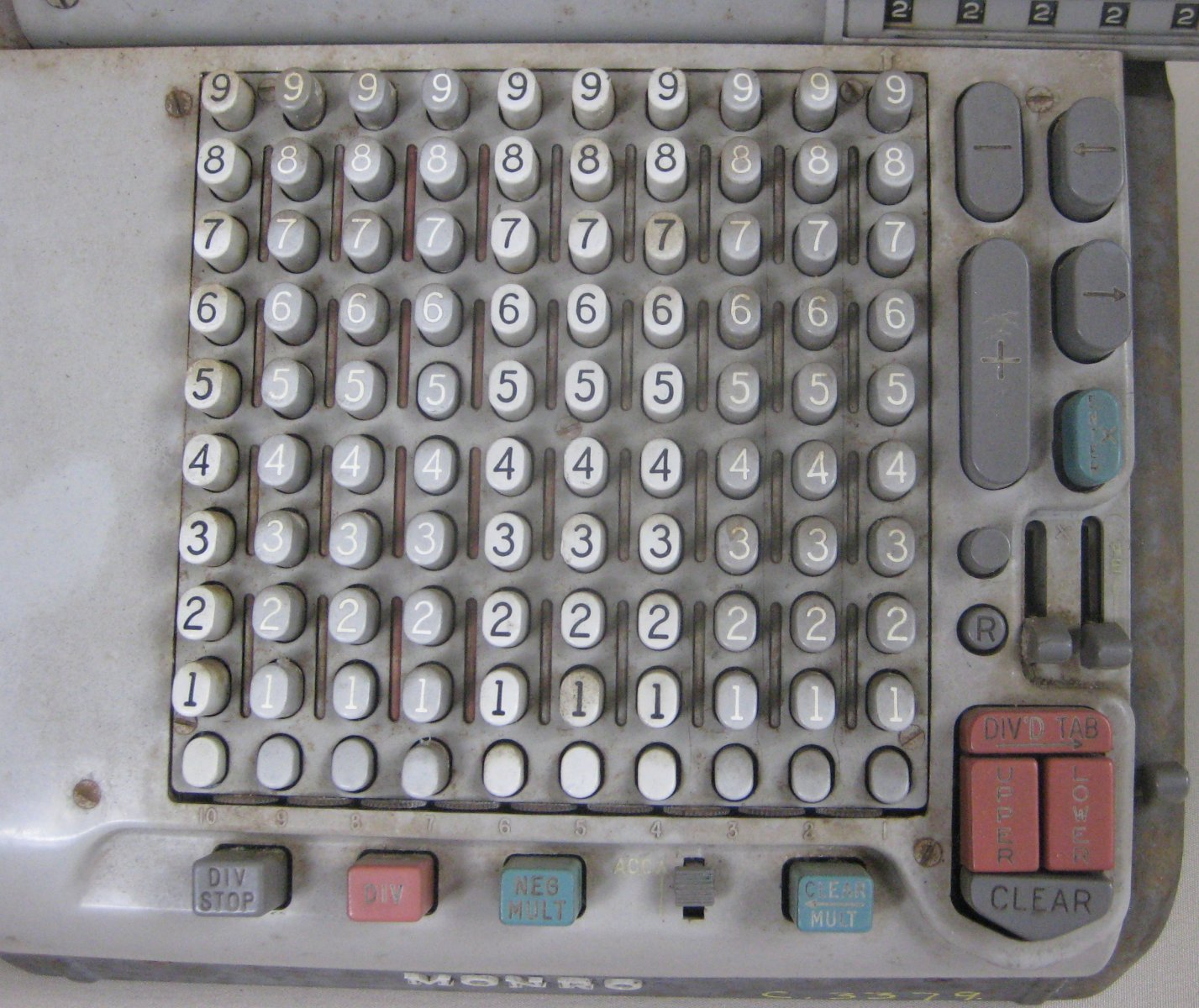•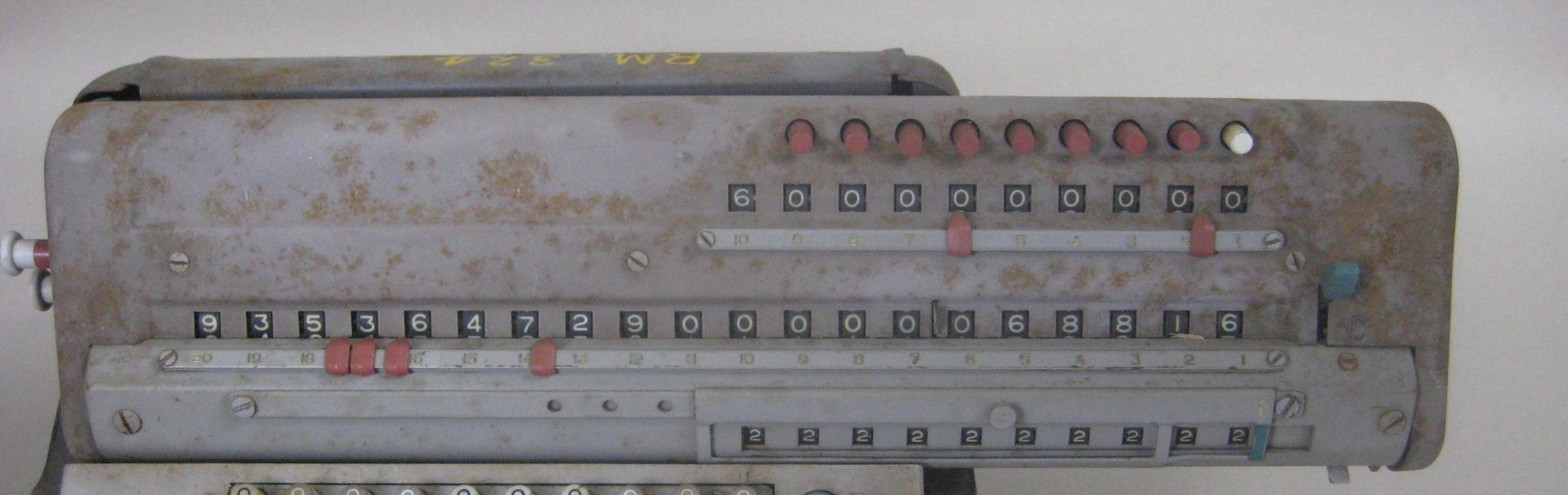•